Courses

# RD Sharma Solutions (Part - 3)- Ex-20.2, Mensuration - I, Class 7, Math Class 7 Notes | EduRev

## Class 7: RD Sharma Solutions (Part - 3)- Ex-20.2, Mensuration - I, Class 7, Math Class 7 Notes | EduRev

The document RD Sharma Solutions (Part - 3)- Ex-20.2, Mensuration - I, Class 7, Math Class 7 Notes | EduRev is a part of the Class 7 Course RD Sharma Solutions for Class 7 Mathematics.
All you need of Class 7 at this link: Class 7

#### QUESTION 12:

There is a rectangular field of size 94 m × 32 m. Three roads each of 2 m width pass through the field such that two roads are parallel to the breadth of the field and the third is parallel to the length. Calculate: (i) area of the field covered by the three roads (ii) area of the field not covered by the roads.

Let ABCD be the rectangular field.
Here,
Two roads which are parallel to the breadth of the field KLMN and EFGH with width 2 m each.
One road which is parallel to the length of the field PQRS with width 2 m.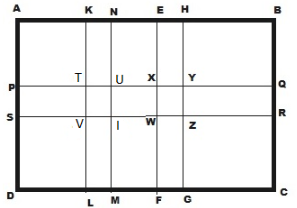Length of the rectangular field AB = 94 m and breadth of the rectangular field BC = 32 m
∴ Area of the rectangular field = Length x Breadth = 94 m x 32 m = 3008 m2
Area of the road KLMN = 32 m x 2 m = 64 m2
Area of the road EFGH = 32 m x 2 m = 64 m2
Area of the road PQRS = 94 m x 2 m = 188 m2

Clearly area of TUVI and WXYZ is common to these three roads.
Thus,
Area of TUVI = 2 m x 2 m = 4 m2
Area of WXYZ = 2 m x 2 m = 4 m2
Hence,
(i) Area of the field covered by the three roads:
= Area (KLMN) + Area (EFGH) + Area (PQRS) − {Area (TUVI ) + Area (WXYZ)}
= [ 64+ 64  + 188 − (4 + 4  )] m2
= 316 m2 − 8 m2
= 308 m2
(ii) Area of the field not covered by the roads:
= Area of the rectangular field ABCD − Area of the field covered by the three roads
= 3008 m2 − 308 m2
= 2700 m2

#### QUESTION 13:

A school has a hall which is 22 m long and 15.5 m broad. A carpet is laid inside the hall leaving all around a margin of 75 cm from the walls. Find the area of the carpet and the area of the strip left uncoverd. If the width of the carpet is 82 cm, find the cost at the rate of Rs 18 per metre.

We have,
Length of the hall PQ = 22 m and breadth of the hall QR = 15.5 m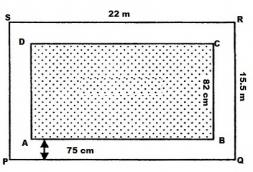∴ Area of the school hall PQRS = 22 m x 15.5 m = 341 m2
Length of the carpet AB = 22 m − ( 0.75 m + 0.75 m) = 20.5 m         [ Since 100 cm = 1 m]
Breadth of the carpet BC = 15.5 m − ( 0.75 m + 0.75 m) = 14 m
∴ Area of the carpet ABCD = 20.5 m x 14 m = 287 m2
Area of the strip = Area of the school hall PQRS − Area of the carpet ABCD
= 341 m2 − 287 m2
= 54 m2
Again,
Area of the 1 m length of carpet = 1 m x 0.82 m = 0.82 m2
Thus,
Length of the carpet whose area is 287 m2 = 287 m2 ÷ 0.82 m2 = 350 m
Cost of the 350 m long carpet = Rs. 18 x 350 = Rs. 6300

#### QUESTION 14:

Two cross roads, each of width 5 m, run at right angles through the centre of a rectangular park of length 70 m and breadth 45 m parallel to its sides. Find the area of the roads. Also, find the cost of constructing the roads at the rate of Rs 105 per m2.

Let ABCD be the rectangular park then EFGH and IJKL the two rectangular roads with width 5 m.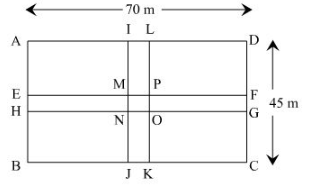Length of the rectangular park AD = 70 cm
Breadth of the rectangular park CD = 45 m
∴ Area of the rectangular park = Length x Breadth = 70 m x 45 m = 3150 m2
Area of the road EFGH = 70 m x 5 m = 350 m2
Area of the road JKIL = 45 m x 5 m = 225 m2
Clearly area of MNOP is common to the two roads.
Thus, Area of MNOP = 5 m x 5 m = 25 m2
Hence,
Area of the roads = Area (EFGH) + Area (JKIL) − Area (MNOP)
= (350  + 225 )  m2− 25 m2 = 550 m2
Again, it is given that the cost of constructing the roads = Rs. 105 per m2
Therefore,
Cost of constructing 550 m2 area of the roads = Rs. (105 × 550)
= Rs. 57750.

#### QUESTION 15:

The length and breadth of a rectangular park are  in the ratio 5 : 2. A 2.5 m wide path running all around the outside the park has an area 305 m2. Find the dimensiions of the park.

We have,
Area of the path = 305 m2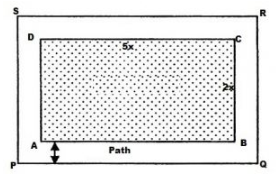Let the length of the park be 5x m and the breadth of the park be 2x m
Thus,
Area of the rectangular park = 5x x 2x = 10x2 m2
Width of the path = 2.5 m
Outer length PQ = 5x m + 2.5 m + 2.5 m (5x + 5) m
Outer breadth QR = 2x + 2.5 m + 2.5 m (2x + 5) m
Area of PQRS = (5x + 5) m x (2x + 5) m = (10x2 + 25x + 10x + 25) m2= (10x2 + 35x + 25) m2
∴ Area of the path = [(10x2 + 35x + 25) − 10x2 ] m2
⇒  305 = 35x + 25
⇒ 305 − 25 = 35x
⇒ 280 = 35x
⇒ x = 280 ÷ 35 = 8
Therefore,
Length of the park = 5x = 5 x 8 = 40 m
Breadth of the park = 2x = 2 x 8 = 16 m

#### QUESTION 16:

A square lawn is surrounded by a path 2.5 m wide. If the area of the path is 165 m2, find the area of the lawn.

Let the side of the lawn be m.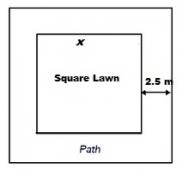Given that width of the path = 2.5 m
Side of the lawn including the path = (x + 2.5 + 2.5) m = (x + 5 ) m
So, area of lawn = (Area of the lawn including the path) − (Area of the path)
We know that the area of a square = (Side)2
∴ Area of lawn  (x2 ) = (x + 5)2 − 165
⇒ x2  = (x2 + 10x + 25) − 165
⇒ 165 = 10x + 25
⇒ 165 − 25  = 10x
⇒ 140 = 10x
Therefore x = 140 ÷ 10 = 14
Thus the side of the lawn = 14 m
Hence,
The area of the lawn = (14 m)2 = 196 m2

The document RD Sharma Solutions (Part - 3)- Ex-20.2, Mensuration - I, Class 7, Math Class 7 Notes | EduRev is a part of the Class 7 Course RD Sharma Solutions for Class 7 Mathematics.
All you need of Class 7 at this link: Class 7Use Code STAYHOME200 and get INR 200 additional OFF Use Coupon Code
All Tests, Videos & Notes of Class 7: Class 7

### Top Courses for Class 7## RD Sharma Solutions for Class 7 Mathematics

97 docs

### Top Courses for Class 7Track your progress, build streaks, highlight & save important lessons and more!

,

,

,

,

,

,

,

,

,

,

,

,

,

,

,

,

,

,

,

,

,

,

,

,

,

,

,

,

,

,

;